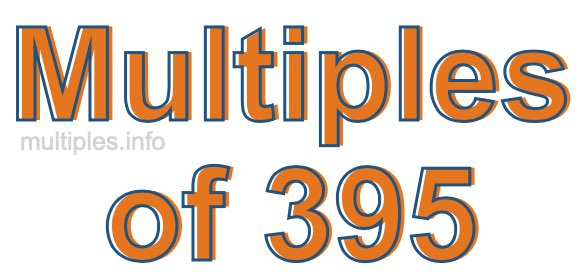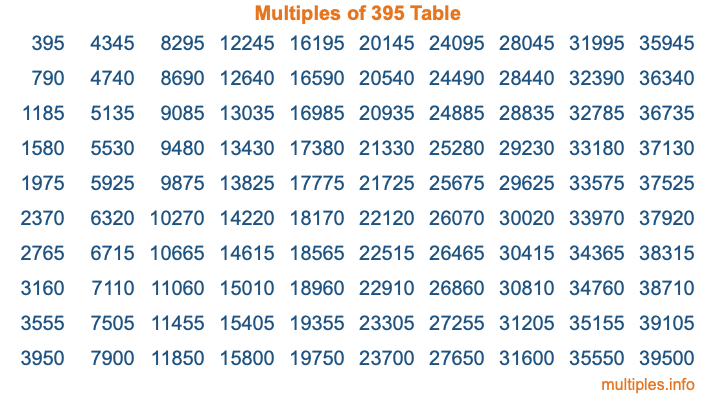Multiples of 395Welcome to the Multiples of 395 page. Here we will first teach you everything you will ever need to know about the multiples of 395, and then give you a study guide summary of everything we taught you to make sure you remember it all. Use this page to look up facts and learn information about the multiples of 395. This page will make you a multiples of three hundred ninety-five expert!

Definition of Multiples of 395
Multiples of 395 are all the numbers that when divided by 395 equal an integer. Each of the multiples of 395 are called a multiple. A multiple of 395 is created by multiplying 395 by an integer.

Therefore, to create a list of multiples of 395, you start with 1 multiplied by 395, then 2 multiplied by 395, then 3 multiplied by 395, and so on for as long as you want. Thus, the list of the first five multiples of 395 is 395, 790, 1185, 1580, and 1975. To see a larger list of multiples of 395, see the printable image of Multiples of 395 further down on this page. We also have a category where you can choose any nth multiple of 395.

Multiples of 395 Checker
The Multiples of 395 Checker below checks to see if any number of your choice is a multiple of 395. In other words, it checks to see if there is any number (integer) that when multiplied by 395 will equal your number. To do that, we divide your number by 395. If the the quotient is an integer, then your number is a multiple of 395.

Is  a multiple of 395?

Least Common Multiple of 395 and ...
A Least Common Multiple (LCM) is the lowest multiple that two or more numbers have in common. This is also called the smallest common multiple or lowest common multiple and is useful to know when you are adding our subtracting fractions. Enter one or more numbers below (395 is already entered) to find the LCM.

Check out our LCM Calculator if you need more details about the Least Common Multiple or if you need the LCM for different numbers for adding and subtraction fractions.

nth Multiple of 395
As we stated above, 395 is the first multiple of 395, 790 is the second multiple of 395, 1185 is the third multiple of 395, and so on. Enter a number below to find the nth multiple of 395.

th multiple of 395

Multiples of 395 vs Factors of 395
395 is a multiple of 395 and a factor of 395, but that is where the similarities end. All postive multiples of 395 are 395 or greater than 395. All positive factors of 395 are 395 or less than 395.

Below is the beginning list of multiples of 395 and the factors of 395 so you can compare:

Multiples of 395: 395, 790, 1185, 1580, 1975, etc.

Factors of 395: 1, 5, 79, 395

As you can see, the multiples of 395 are all the numbers that you can divide by 395 to get a whole number. The factors of 395, on the other hand, are all the whole numbers that you can multiply by another whole number to get 395.

It's also interesting to note that if a number (x) is a factor of 395, then 395 will also be a multiple of that number (x).

Multiples of 395 vs Divisors of 395
The divisors of 395 are all the integers that 395 can be divided by evenly. Below is a list of the divisors of 395.

Divisors of 395: 1, 5, 79, 395

The interesting thing to note here is that if you take any multiple of 395 and divide it by a divisor of 395, you will see that the quotient is an integer.

Multiples of 395 Table
Below is an image of the first 100 multiples of 395 in a table. The table is in chronological order, column by column. The first column has the first ten multiples of 395, the second column has the next ten multiples of 395, and so on.The Multiples of 395 Table is also referred to as the 395 Times Table or Times Table of 395. You are welcome to print out our table for your studies.

Negative Multiples of 395
Although not often discussed or needed in math, it is worth mentioning that you can make a list of negative multiples of 395 by multiplying 395 by -1, then by -2, then by -3, and so on, to get the following list of negative multiples of 395:

-395, -790, -1185, -1580, -1975, etc.

Multiples of 395 Summary
Below is a summary of important Multiples of 395 facts that we have discussed on this page. To retain the knowledge on this page, we recommend that you read through the summary and explain to yourself or a study partner why they hold true.

There are an infinite number of multiples of 395.

A multiple of 395 divided by 395 will equal a whole number.

395 divided by a factor of 395 equals a divisor of 395.

The nth multiple of 395 is n times 395.

The largest factor of 395 is equal to the first positive multiple of 395.

395 is a multiple of every factor of 395.

395 is a multiple of 395.

A multiple of 395 divided by a divisor of 395 equals an integer.

395 divided by a divisor of 395 equals a factor of 395.

Any integer times 395 will equal a multiple of 395.

Multiples of a Number
Here you can get the multiples of another number, all with the same attention to detail as we did for multiples of 395 on this page.

Multiples of
Multiples of 396
Did you find our page about multiples of three hundred ninety-five educational? Do you want more knowledge? Check out the multiples of the next number on our list!